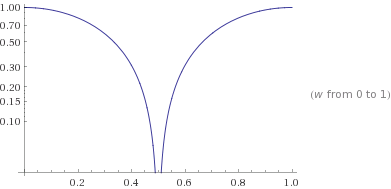## Sunday, January 31, 2016

### The Boxcar Digital Lowpass Filter

In embedded systems it is quite common to need a little bit of filtering on analog quantities. Programmers sometimes feel they need the filtering to help smooth any analog conversion noise. Two type of filters commonly used are the Boxcar and the Exponential filter. Both are simple to implement. Its important to understand the impact these filters have on delay and frequency response, and to evaluate their comparative effectiveness.

The Boxcar filter is a simple averaging filter. Technically it is an FIR filter. Typically you would obtain your next analog value, add it to the last n-1 values read, then divide by n. It's just a simple average of the last few values read.

A common value for n is 4. The Z transform of a filter with n=4 is H(z) = ¼ (z3+z2+z+1)/(z3) . There are three poles at the origin in the Z domain. Thus this filter is stable. There is one real zero at -1, and two complex conjugate zero's at +i and -i. The (normalized) frequency response is given by H(e) = magnitude(¼ + ¼e-jω+ ¼e-j2ω+¼e-j3ω).
Click on the link to use Wolfram-Alpha to plot this function. In the following image (copied from Wolfram) we see several cycles of normalized frequency response including mirroring above half the sample rate.   The mirroring is how aliasing shows up in the frequency response.Box Car Filter Normalized Response.  N=4.

Note that the horizontal axis is in Hz. The sampling frequency is 1Hz.  (The response is normalized to 1 Hz.)  One half the sample rate is 0.5Hz - the Nyquist rate. What you are seeing above 0.5Hz is a periodic mirror of the response. But lets focus on the response below 0.5 Hz.  How UGLY for a low pass response.  Lets try to plot the response for n=2. With this filter all we do is add the current and previous samples and divide by 2. The characteristic equation is Y = ½(X + Xz-1) .  The transfer function is H(z) = ½(1+z-1) = ½(1+z)/z . This has a single pole at 0 and a zero at -1. Thus its stable. The normalized frequency domain function H(e) = ½(1+e-jω).  Its normalized for a sample time of 1sec. Click here to have Wolfram Alpha plot the response.Box Car Filter Normalized Response.  N=2.
This looks more like a low pass response. Note that the horizontal axis this time is in Hz. Since the sample time is a normalized 1second, then the sample frequency is 1.0 Hz, and the Nyquist frequency is 0.5 Hz.

In my next Laboratory Note we will look at the Exponential Filter. This is actually my favorite and I will show you why!

## Sunday, January 24, 2016

### 3D web graphics.

A new feature of modern web browsers is web.gl.  This language allows browsers to take advantage of the hardware graphics acceleration in your computer.  Its a little awkward to program in so the three.js library was born.  Its an open source project.  To learn this framework I created some experiments.  Please click on the links below.  The images can be rotated and panned with your mouse.

A starburst pattern   A cylinder made of lines
A flat Square   An open cube with shading
Polygon with gradient coloring - these were some simple, first experiments

Stairway with Shading       Mesh a Cylinder
Mesh a segmented Cylinder
Mesh a Cylinder turned into a vase
Mesh a Cylinder turned into a torus - these are meshed from many tiny, individual
triangles. They do not use the Three.js premeshed objects.

Cube matrix with animated color    An animated solar system
Bunch of Boxes   A ziggurat
A ziggurat with controls
A helix of objects  A helix of any 'ole object

Citi-scape
Cubes changing color      A tiny solar system
A torus explorer   Click square to make wave

Finally, these are some water simulations.  Click on the water to make waves.:
Hugo Elias Algorithm - makes multiple waves with interference at edges
Transverse Wave Algorithm - can make 1 wave at a time.
Another algorithm